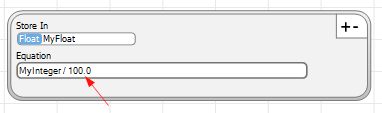Seleccionar página

# Calculator

 Calculator result is stored in this Tag. Make sure Tag is of data type large enough for result. Your equation goes here. Type in it, or use the buttons below to create. Tag values can be used in equations. Select Tag here, then press insert to add to equation. In equation, start first with * operations, then, with / 0x : Specifies that number to follow is Hexadecimal. [ ] : Used to index Tag Array itemsYou can also us it for binary tags (manually enter), but the result must be inside a no binary tag. The example here, is : InBitC1 OR InBitC2 XOR (NOT InBitC3) AND InBitC4If you want to divide an integer by a number and get a float as the result, add the decimal point :